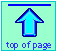Note: this document will print in an appropriately modified format (9 pages)

1  What is electrolysis?Electrolysis refers to the decomposition of a substance by an electric current. The electrolysis of sodium and potassium hydroxides, first carried out in 1808 by Sir Humphrey Davey, led to the discovery of these two metallic elements and showed that these two hydroxides which had previously been considered un-decomposable and thus elements, were in fact compounds.

Electrolysys is carried out in electrochemical cells which are forced to operate in the "reverse", or non-spontaneous direction. The free energy is supplied in the form of electrical work done on the system by the outside world (the surroundings). This is the only fundamental difference between an electrolytic cell and the galvanic cell in which the free energy supplied by the cell reaction is liberated as heat released or work done on the surroundings.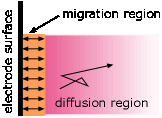A common misconception about electrolysis is that "ions are attracted to the oppositely-charged electrode." This is true only in the very thin interfacial region near the electrode surface. Ionic motion throughout the bulk of the solution occurs mostly by diffusion, which is the transport of molecules in response to a concentration gradient. Migration— the motion of a charged particle due to an applied electric field, is only a minor player, producing only about one non-random jump out of around 100,000 random ones for a 1 volt cm–1 electric field. Only those ions that are near the interfacial region are likely to undergo migration.

### Electrolysis of molten salts

Many of the metallic elements commonly occur in nature as oxide, sulfide, or carbonate ores, which must undergo smelting to release the metal.  Of course, any compound will decompose into its elements if heated to a sufficiently high temperature. For most metals, the required temperature is too high to make this economically practical. For most metals, chemical reduction is the preferred method of smelting. Thus iron, the most important structural metal, is produced by heating the ore in the presence of carbon monoxide, which reduces FeO and related oxides to the metal by stripping the oxygen from the ore. A few ores, such as HgS, can be smelted by simple heating, a process known as roasting.

Although electrolytic reduction of these elements is possible, it is rarely practical, owing to the high temperatures required to melt the ores.

For most metals of Groups 1 and 2, the situation is quite different; electrolysis of their molten salts turns out to be the only practical method of production.  The same is true of aluminum, whose industrial production by electrolysis is described near the end of this document.

Davey's 1808 discovery served for many years as the basis for the 1888 Castner process for the commercial production of metallic sodium.

 cathode: 2Na+ + 2 e– → 2 Na(l) anode: 2 OH– → ½ O2(g) + H2O + 2e– net: 2Na+ + 2OH– →2 Na(s) + O2(g)

Why use electrolysis here? The fact that NaOH is a stable salt implies that its decomposition back into its elements is a highly endothermic process. Electrolysis is the most efficient means of supplying the required energy.

In modern times, the Castner process has been displaced by the more-efficient Downs process. Electrolysis of molten alkali halides is the usual industrial method of preparing all of the alkali metals:

 cathode: Na+ + e– → Na(l) anode: Cl– → ½ Cl2(g) + e– net: Na+ + Cl– → Na(s) + ½ Cl2(g)

Thus if a solution of nickel chloride undergoes electrolysis at platinum electrodes, the reactions are

 cathode: Ni2+ + 2 e– → Ni(s) E° = –0.24 v anode: 2 Cl– → Cl2(g) + 2 e– E° = –1.36 v net: Ni2+ + 2 Cl– → Ni(s) + Cl2(g) E° = –1.60 v

2  Electrolysis in aqueous solutions

Water is the chemist's most common and versatile solvent, but it can be a complicating factor in electrochemistry because it is itself capable of undergoing both oxidation, releasing oxygen at the anode,

H2O → ½ O2(g) + 2 H+ + 2 e      E° = –1.23 v

and reduction, producing hydrogen gas at the cathode.

2 H2O + 2 e → H2(g) + 2 OH      E° = –0.83 v

Thus if an aqueous solution is subjected to electrolysis, one or both of the above reactions may be able to compete with the electrolysis of the solute. However, although both of the above reactions can be thermodynamically allowed at commonly used applied voltages, they are kinetically inhibited owing to the high activation energies associated with the liberation of gases at electrodes. In order to force these reactions to take place, an additional potential, known as an overvoltage, must be supplied. The magnitude of these overvoltages varies with current and also with the composition of the electrode, and is frequently high enough to inhibit the gas discharge.

Thus a solution of nickel chloride can undergo electrolysis at platinum electrodes without evolution of oxygen, even though the applied potential required to oxidize the chlorine theoretically allows it:

 cathode: Ni2+ + 2 e– → Ni(s) E° = –0.24 v anode: 2 Cl– → Cl2(g) + 2 e– E° = –1.36 v net: Ni2+ + 2 Cl– → Ni(s) + Cl2(g) E° = –1.60 v

In contrast, if we try to electrolyze a solution of sodium chloride at most electrodes, hydrogen is produced at the cathode instead of sodium:

 cathode: 2 H2O + 2 e– → H2(g) + 2 OH– E =+0.41 v  ([OH–] = 10—7 M) anode: Cl– → ½ Cl2(g) + e– E° = –1.36 v net: Cl– + H2O → 2 H2(g) + ½ Cl2(g) + 2 OH– E = –0.95 v

This turns out to be quite fortunate; electrolysis of salt ("brine") is carried out on a huge scale and is the basis of the chloralkali industry.

Electrolysis of solutions in which the cation is a sulfate or nitrate are similarly immune to the decomposition of these cations.

### Electrolysis of water

Pure water is an insulator and cannot undergo signifigant electrolysis without adding an electrolyte. If the object is to produce hydrogen and oxygen, the electrolyte must be energetically more difficult to oxidize or reduce than water itself. Electrolysis of a solution of sulfuric acid or of a salt such as NaNO3 results in the decomposition of water at both electrodes:cathode: 2 H2O + 2 e– → H2(g) + 2 OH– E° = –0.83 v anode: H2O → ½ O2(g) + 2 H+ + 2 e– E° = –1.23 v net: 3 H2O → H2(g) + ½ O2(g) E = –2.06 v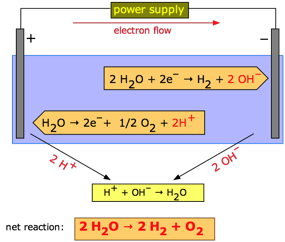Electrolytic production of hydrogen is usually carried out with a dilute solution of sulfuric acid; see here for a concise summary of the chemistry and energetics. This process is generally too expensive for industrial production unless highly pure hydrogen is required. However, it becomes more efficient at higher temperatures, where thermal energy reduces the amount of electrical energy required, so there is now some interest in developing high-temperature electrolytic processes. Most hydrogen gas is manufactured by the steam reforming of natural gas.

One mole of electric charge (96,500 coulombs), when passed through a cell, will discharge half a mole of a divalent metal ion such as Cu2+.

This relation was first formulated by Faraday in 1832 in the form of two laws of electrolysis:

1. The weights of substances formed at an electrode during electrolysis are directly proportional to the quantity of electricity that passes through the electrolyte.

2. The weights of different substances formed by the passage of the same quantity of electricity are proportional to the equivalent weight of each substance.

The equivalent weight of a substance is defined as the molar mass, divided by the number of electrons required to oxidize or reduce each unit of the substance. Thus one mole of V3+ corresponds to three equivalents of this species, and will require three faradays of charge to deposit it as metallic vanadium.

Most stoichiometric problems involving electrolysis can be solved without explicit use of Faraday's laws. The "chemistry" in these problems is usually very elementary; the major difficulties usually stem from unfamiliarity with the basic electrical units:

• current (amperes) is the rate of charge transport; 1 amp = 1 c/sec.
• power (watts) is the rate of energy production or consumption;
1 w = 1 J/sec = 1 volt-amp; 1 watt-sec = 1 J, 1 kw-h = 3600 J.

3  Industrial electrolytic processes

For many industrial-scale operations involving the oxidation or reduction of both inorganic and organic substances, and especially for the production of the more active metals such as sodium, calcium, magnesium, and aluminum, the most cost-effective reducing agent is electrons supplied by an external power source. The two most economically important of these processes are described below.

### The chloralkali industry

The electrolysis of brine is carried out on a huge scale for the industrial production of chlorine and caustic soda (sodium hydroxide). Because the reduction potential of Na+ is much higher than that of water, the latter substance undergoes decomposition at the cathode, yielding hydrogen gas and OH.

Anode reactions Cathode reactions 2 Cl– → Cl2(g) + 2 e–(i) 4 OH– → O2(g) + 2 H2O + 4 e–(ii) –1.36 v –0.40 v Na+ + e– → Na(s)(iii) H2O + 2 e– → H2(g) + 2 OH–(iv) –2.7 v +0.41 v

A comparison of the s would lead us to predict that the reduction (ii) would be favored over that of (i). This is certainly the case from a purely energetic standpoint, but as was mentioned in the section on fuel cells, electrode reactions involving O2 are notoriously slow (that is, they are kinetically hindered), so the anodic process here is under kinetic rather than thermodynamic control. The reduction of water (iv) is energetically favored over that of Na+ (iii), so the net result of the electrolysis of brine is the production of Cl2 and NaOH ("caustic"), both of which are of immense industrial importance:

2 NaCl + 2 H2O → 2 NaOH + Cl2(g) + H2(g)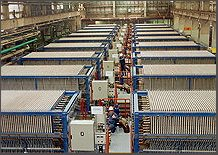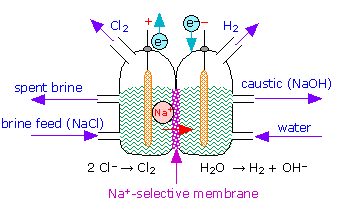A modern industrial chloralkali plant Schematic diagram of a cell for the production of chlorine

Since chlorine reacts with both OH and H2, it is necessary to physically separate the anode and cathode compartments. In modern plants this is accomplished by means of an ion-selective polymer membrane, but prior to 1970 a more complicated cell was used that employed a pool of mercury as the cathode. A small amount of this mercury would normally find its way into the plant's waste stream, and this has resulted in serious pollution of many major river systems and estuaries and devastation of their fisheries.

### Electrolytic refining of aluminum

Aluminum is not easily obtained from its ores, and was in fact considered an exotic and costly metal until the late 19th century.

Aluminum is present in most rocks and is the most abundant metallic element in the earth's crust (eight percent by weight.) However, its isolation is very difficult and expensive to accomplish by purely electrolytic means, owing both to the very high melting point of its oxide and to the high (–1.66 v) of the Al3+/Al couple. This high also prevents the isolation of Al by electrolysis of aqueous solutions of its compounds, since the water would be electrolyzed preferentially.

Most of the earth's accessible aluminum is in the form of its hydrous oxide AlOOH and hydroxide Al(OH)3 which occur within a rock-like ore called bauxite.

The production of aluminum from bauxite begins with the conversion of its aluminum-containing components into aluminum hydroxide Al(OH)3. The hydroxide is then dissolved in hot NaOH to form sodium aluminate NaAlO2followed by a series of steps that eventually result in aluminum oxide Al2O3, commonly known as alumina.

The very high melting point (2072 °C) of alumina made the electrolysis of molten oxide impractical until 1886, when Charles Hall (U.S.A) and Paul Hérault (France) independently invented workable process.

The Hall-Hérault process takes advantage of the principle that the melting point of a substance is reduced by admixture with another substance with which it forms a homogeneous phase. Instead of using the pure alumina ore Al2O3 which melts at 2050°C, it is mixed with cryolite, which is a natural mixture of NaF and AlF3, thus reducing the temperature required to a more manageable 1000°C.Charles HallPaul Hérault

The anodes of the cell are made of carbon (actually a mixture of pitch and coal), and this plays a direct role in the process; the carbon gets oxidized (by the oxide ions left over from the reduction of Al3+ to CO, and the free energy of this reaction helps drive the aluminum reduction, lowering the voltage that must be applied and thus reducing the power consumption. This is important, because aluminum refining is the largest consumer of industrial electricity, accounting for about 5% of all electricity generated in North America. Since aluminum cells commonly operated at about 100,000 amperes, even a slight reduction in voltage can result in a large saving of power.

The net reaction is

2 Al2O3 + 3 C → 4 Al + 3 CO2

However, large quantities of CO and of HF (from the cryolite), and hydrocarbons (from the electrodes) are formed in various side reactions, and these can be serious sources of environmental pollution.